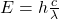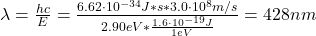PLEASE HELP ME WITH THIS ONE QUESTION A photon has 2.90 eV of energy. What is the photon’s wavelength? (h = 6.626 x 10^-19, 1 eV = 1.

Question

A photon has 2.90 eV of energy. What is the photon’s wavelength? (h = 6.626 x 10^-19, 1 eV = 1.6 x 10^-19 J)

A) 677 nm

B) 218 nm

C) 345 nm

D) 428 nm

in progress 0
6 months 2021-07-26T08:47:17+00:00 1 Answers 4 views 0

The correct option is D.

Explanation:

The wavelength of the photon can be calculated with the following equation:Where:

E: is the energy of the photon = 2.90 eV

h: is the Planck’s constant = 6.62×10⁻³⁴ J.s

c: is the speed of light = 3×10⁸ m/s

λ: is the wavelength

Hence, the photon’s wavelength is:Therefore, the correct option is D.

I hope it helps you!